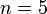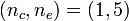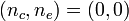# User:Tohline/Appendix/Ramblings

## Ramblings

Sometimes I explore some ideas to a sufficient depth that it seems worthwhile for me to archive the technical derivations even if the idea itself does not immediately produce a publishable result. This page, which has a simple outline layout, provides links to these various pages of technical notes.

1. Relationship between HNM82 models and T1 coordinates
2. Orthogonal Curvilinear Coordinates
3. Playing with the Spherical Wave Equation
4. Analyzing Azimuthal Distortions
5. Integrals of Motion
1. Old discussion
2. T3 Coordinates
1. Special (quadratic) case: Joel's Derivation vs. Jay's Derivation
3. Killing Vector Approach; Jay Call's related Talk page
4. Characteristic Vector for T3 Coordinates
5. T4 Coordinates (Abandoned by Joel 7/6/2010 because non-orthogonal)
7. Photosphere of Stably Accreting DWD
8. Initial Effort to Explain Jay Call's Hybrid Scheme in the Context of Zach Byerly's Dissertation
9. Exploring the Properties of Radial Oscillations in Pressure-Truncated n = 5 Polytropes
10. Instabilities Associated with Equilibrium Sequence Turning Points
11. Derivations Related to Ledoux's Variational Principle
12. More on Zero-Zero Bipolytropes
13. Analyzing Five-One Bipolytropes
14. On the Origin of Planetary Nebulae (Investigation Resulting from a July, 2013 Discussion with Kundan Kadam)</lli>
15. Looking outward, from Inside a Black Hole
16. Radial Dependence of the Strong Nuclear Force
17. Dyson (1893a) Part I:  Some Details
19. Saturn
20. Doctoral students Tohline has advised over the years

## Mathematics

1. Roots of Cubic Equation
1. PP Tori — Also includes cube root of a complex number
2. Srivastava's F-Type solution for$~n=5$ polytropes.
3. Murphy & Fiedler's Bipolytrope with$~(n_c, n_e) = (1,5)$
4. Analytic Eigenfunctions for Bipolytropes with$~(n_c, n_e) = (0, 0)$ — also involves cube root of a complex number
2. Roots of Quartic Equation
1. Analytic Eigenfunction for Bipolytropes with$~(n_c, n_e) = (0, 0)$
2. Determine temperature from total pressure
3. Singular Sturm-Liouville (eigenvalue) Problem
1. Oscillations of PP Tori in the slim torus limit
2. Characteristics of unstable eigenvectors in self-gravitating tori
4. Approximate Power-Series Expressions
5. Fourier Series
6. Special Functions & Other Broadly Used Representations
7. Green's Function in terms of Toroidal Functions
1. Compact Cylindrical Green Function
2. Toroidal configurations & related coordinate systems — Includes EUREKA! moment; also uses wikitable overflow (scrolling) box
3. Toroidal Coordinate Integration Limits$~\Leftarrow ~~$ Includes Table of Example K(k) and E(k) Function Values
4. Using Toroidal Coordinates to Determine the Gravitational Potential (Initial Presentation)
5. Relationships between Toroidal Functions$~\Leftarrow ~~$ 5 plots of [MF53] data included here
6. Confusion Regarding Whipple Formulae
7. Pulling It All Together$~\Leftarrow ~~$ 2 additional plots of [MF53] data included here

## Computer-Generated Holography

 © 2014 - 2020 by Joel E. Tohline |   H_Book Home   |   YouTube   | Appendices: | Equations | Variables | References | Ramblings | Images | myphys.lsu | ADS |